• 算术运算 ...包括：加法、减法、乘法、除法、乘方、开方等几种运算形式。 其中加减为一级运算，乘除为二级运算，乘方、开方为三级运算。在一道算式中，如果有几级运算存在，则应先进行高级运算，再进行...
什么是算术运算？什么是关系运算？什么是逻辑运算？
【答案解析】
算术运算：
算术运算即“四则运算”，是加法、减法、乘法、除法、乘方、开方等几种运算的统称。  其中加减为一级运算，乘除为二级运算，乘方、开方为三级运算。在一道算式中，如果有多级运算存在，则应先进行高级运算，再进行低一级的运算。  C语言中的算熟运算符包括：+、-、*、/、++、--、% 等种类。  如果只存在同级运算；则从左至右的顺序进行；如果算式中有括号，则应先算括号里边，再按上述规则进行计算。
示例：$(1 + 1)^{2} * 4+5 * 3$
解析：
先进行括号内运算1+1，然后进行乘方运算得到结果4.接下来与4相乘，得到结果16因为乘法优先级大于加法，因此先进行5*3，得到结果15最终相加得到结果31
结果：31
关系运算：
关系的基本运算有两类：一类是传统的集合运算（并、差、交等），另一类是专门的关系运算（选择、投影、连接、除法、外连接等），而在C语言中，关系运算通常被认为是比较运算，将两个数值进行比较，判断比较结果是否符合给定的条件。  常见的关系运算符包括：<、<=、>、>=、==、!= 等种类。  其中，前4种关系运算符(<、<=、>、>= )的优先级别相同，后2种(==、!=)也相同。而前4种高于后2种。  例如, > 优先于 == 。而 > 与 < 优先级相同。 并且，关系运算符的优先级低于算术运算符，关系运算符的优先级高于赋值运算符(=)。
逻辑运算：
在逻辑代数中，有与、或、非三种基本逻辑运算。表示逻辑运算的方法有多种，如语句描述、逻辑代数式、真值表、卡诺图等。而在C语言中，逻辑运算通常用于使用逻辑运算符将关系表达式或其它逻辑量连接起来组成逻辑表达式用来测试真假值。  常见的逻辑运算符包括：&&、||、! 等种类  &&： 与是双目运算符，要求有两个运算对象，表示两个运算对象都成立，则结果为真，否则结果为假。
例如：(a<b) && (x>y)，表示(a<b)和(x>y)同时成立则为真。
||：是双目运算符，要求有两个运算对象，表示两个运算对象只要任意一个成立，则结果为真，否则结果为假。  例如：(a<b) && (x>y)，表示(a<b)和(x>y)两个对象中任意一个成立则结果为真。  !：是单目运算符，只要求有一个运算对象，表示取运算对象反义，运算对象为真则结果为假，运算对象结果为假则结果为真。  例如：!(a>b)，表示(a>b)成立时结果为假，不成立时结果为真。  若在一个逻辑表达式中包含多个逻辑运算符，则优先次序为： ! > && > ||。当然若一个逻辑表达式中包含括号括起来的子逻辑，则优先括号内的子逻辑判断。
示例：
(1>2)||(2>3)&&(4>3) 结果为0 !(1>2)||(2>3)&&(4>3)结果为1  注：&&优先级大于||，((2>3)&&(4>3))无法同时成立，则结果为假，然后与(1>2)结果进行逻辑或运算，两者都为假因此第一次结果为假。 而第二次!优先级最高，先对(1>2)的结果取逻辑非，得到结果为真，因此结果为真。 
展开全文• 关系代数是一抽象的查询语言，用对关系运算来表达查询，作为研究关系数据语言的数学工具。 基本的关系代数算法： 传统的集合运算：并、交、差、广义笛卡尔积； 专门的集合运算：选择、投影、象集、连接（等值...

关系代数是一种抽象的查询语言，用对关系的运算来表达查询，作为研究关系数据语言的数学工具。1

目录
基本的关系代数算法传统的集合运算并

∪

\cup

交

∩

\cap

差

−

-

笛卡尔积（广义）

×

\times

专门的集合运算选择

σ

\sigma

投影

π

\pi

象集（选择+投影）
连接

⋈

\bowtie

等值连接自然连接
除

÷

\div

Refences

基本的关系代数算法
传统的集合运算
并

∪

\cup

要求：参与并运算的两张关系表必须具有相同的属性（列名）；运算方法：选取R、S关系表中的所有元组合并，建立新表；表达式：

R

∪

S

=

{

i

∣

i

∈

R

∨

i

∈

S

}

.

R \cup S = \{ i|i \in R \vee i \in S\}.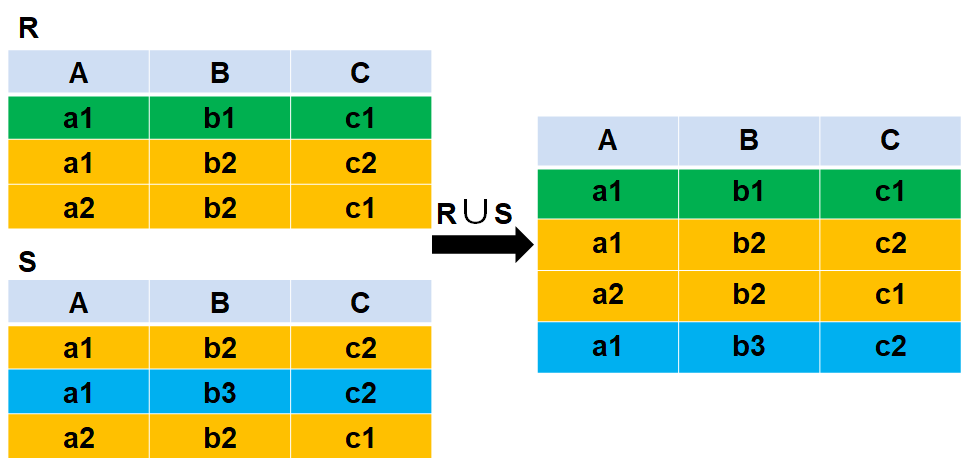交

∩

\cap

要求：参与交运算的两张关系表必须具有相同的属性（列名）；运算方法：选取R、S关系表中的所有相同元组，建立新表；表达式：

R

∩

S

=

{

i

∣

i

∈

R

∧

i

∈

S

}

.

R \cap S = \{ i | i \in R \wedge i \in S \}.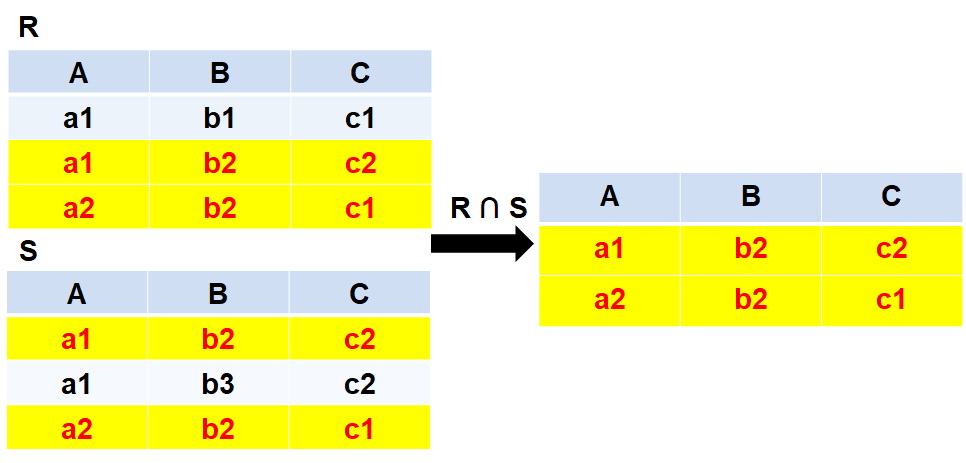差

−

-

要求：参与交运算的两张关系表必须具有相同的属性（列名）；运算方法：选取R表中与S表中任意元组不同的元组，建立新表；表达式：

R

−

S

=

{

i

∣

i

∈

R

∧

i

∉

S

}

.

R - S = \{ i | i \in R \wedge i \notin S \}.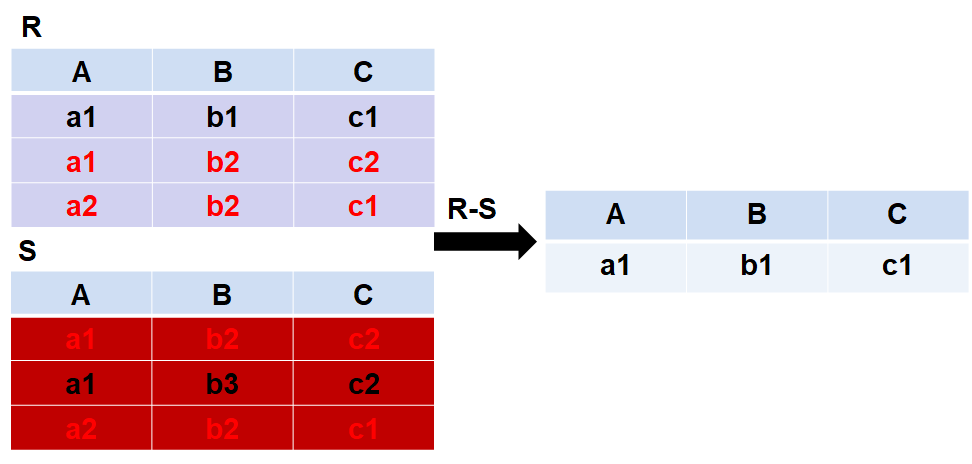笛卡尔积（广义）

×

\times

要求：R表和S表可以拥有不同的属性（列）；运算方法：R的每一行元组后面都要跟随S的所有元组；表达式：

R

×

S

=

{

i

R

i

S

^

∣

i

R

∈

R

∧

i

S

∈

S

}

.

R \times S = \{ \widehat{i_R i_S} | i_R \in R \wedge i_S \in S \}.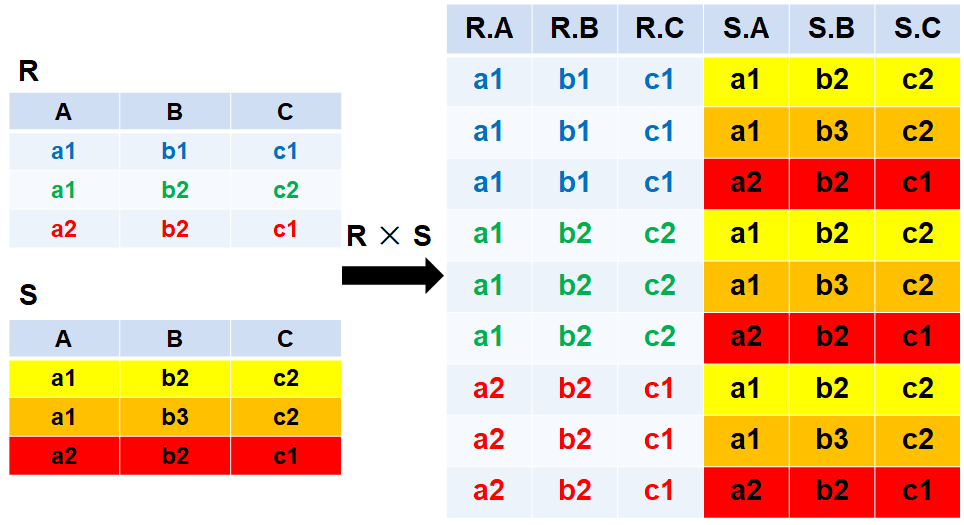专门的集合运算
选择

σ

\sigma

要求：拥有一个基于R表中属性A（列A）的逻辑表达式F；运算方法：从R表中选择符合逻辑表达式F的元组（行），建立新表；表达式：

F

(

i

)

=

i

[

A

]

θ

a

(

θ

为

比

较

运

算

符

,

可

为

>

,

<

,

≥

,

≤

,

=

或

≠

)

,

σ

F

(

R

)

=

{

i

∣

i

∈

R

∧

F

(

i

)

}

.

F(i)=i[A]\,\theta\,a \\ (\theta 为比较运算符,可为>,<,\geq,\leq,=或\neq),\\ \sigma_F(R)= \{ i | i \in R \wedge F(i)\}.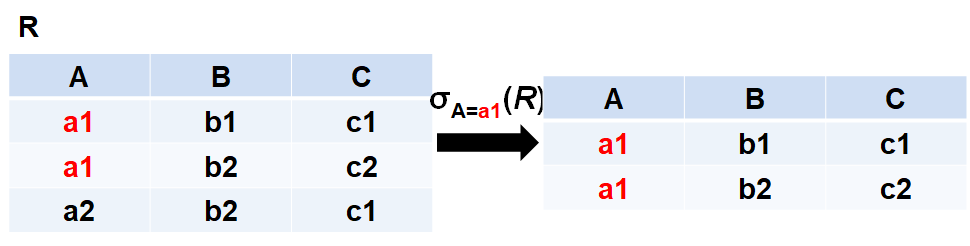投影

π

\pi

要求：所选的属性组A,B必须来源于R表的属性（列名）；运算方法：从R表中选择指定的属性组（列），建立新表；表达式：

π

A

,

B

(

R

)

=

{

i

[

A

,

B

]

∣

i

∈

R

}

.

\pi_{A,B}(R)= \{ i[A,B] | i \in R \}.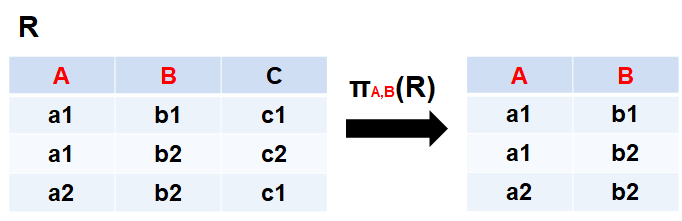象集（选择+投影）
要求：所选的具体属性值a必须来源于R表中存在的属性（列）；运算方法：从R表中选择指定的属性值a所在的行，投影出剩下的其他属性Z，建立新表；表达式：

π

Z

(

σ

A

=

a

(

R

)

)

=

{

i

[

Z

]

∣

i

∈

R

∧

i

[

A

]

=

a

}

.

\pi_{Z}(\sigma_{A=a}(R))= \{ i[Z] | i \in R \wedge i[A]=a \}.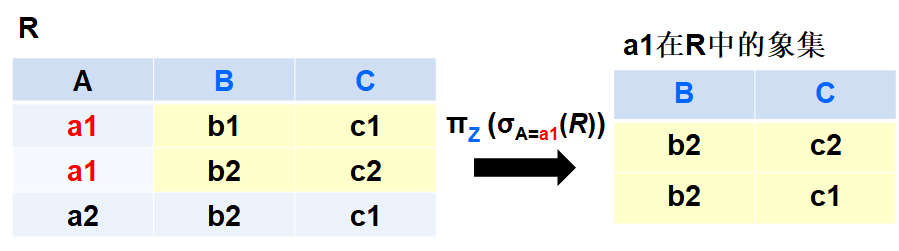连接

⋈

\bowtie

要求：A、B属性组（列）必须分别为R表和S表中的属性，且具有可比性（如皆为日期属性、数值属性等）；运算方法：先使用广义笛卡尔积（见上文）算出R

×

\times

S，再在R

×

\times

S中选取符合逻辑表达式F的元组，建立新表；表达式：

F

(

i

)

=

i

R

[

A

]

θ

i

S

[

B

]

(

θ

为

比

较

运

算

符

,

可

为

>

,

<

,

≥

,

≤

,

=

或

≠

)

,

R

⋈

F

S

=

{

i

R

i

S

^

∣

i

R

∈

R

∧

i

S

∈

S

∧

F

(

i

)

}

.

F(i)=i_R[A] \,\theta\, i_S[B]\\ (\theta 为比较运算符,可为>,<,\geq,\leq,=或\neq),\\ R\mathop{\bowtie}\limits_{F} S=\{\widehat{i_Ri_S} | i_R \in R \wedge i_S \in S \wedge F(i) \}.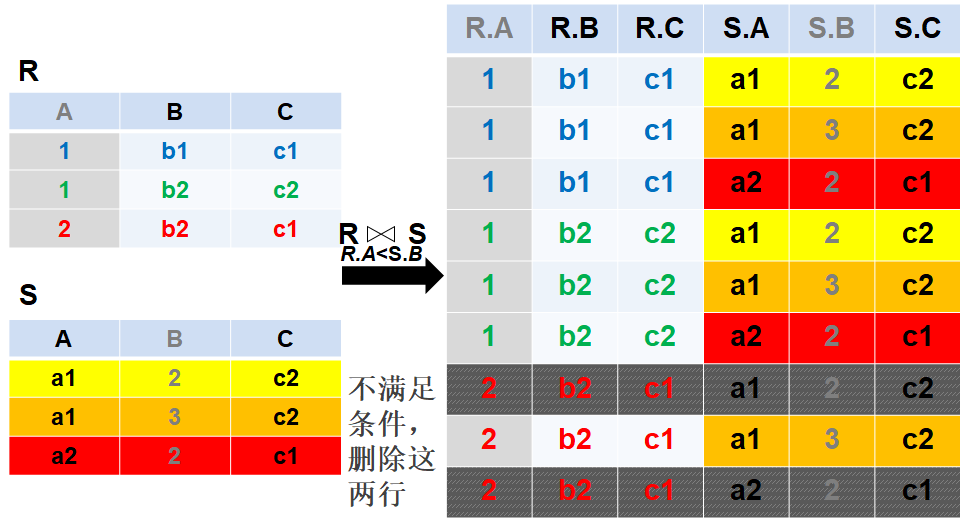等值连接
要求：在满足连接要求的前提下，逻辑表达式F中的

θ

\,\theta\,

为“

=

\,=\,

”；运算方法：先使用广义笛卡尔积（见上文）算出R

×

\times

S，再在R

×

\times

S中选取表R的属性A与表S的属性B值相等的元组，建立新表；表达式：

R

⋈

A

=

B

S

=

{

i

R

i

S

^

∣

i

R

∈

R

∧

i

S

∈

S

∧

i

R

[

A

]

=

i

S

[

B

]

}

.

R\mathop{\bowtie}\limits_{A = B} S=\{\widehat{i_Ri_S} | i_R \in R \wedge i_S \in S \wedge i_R[A] = i_S[B] \}.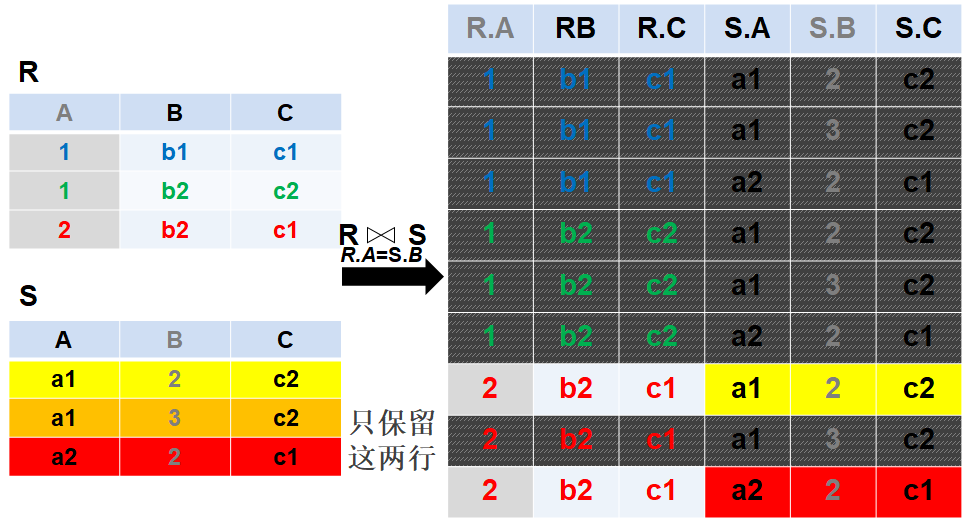自然连接
要求：在满足连接要求的前提下，R表和S表中必须要有至少一个相同的属性C（列C）；运算方法：先使用广义笛卡尔积（见上文）算出R

×

\times

S（即下图中右侧较大的整个表），再在R

×

\times

S中选取两表中属性C值 相等 的元组，建立新表，并删除重复的相同属性组，仅保留一组即可；表达式：

R

⋈

S

=

{

i

R

i

S

^

∣

i

R

∈

R

∧

i

S

∈

S

∧

i

R

[

A

]

=

i

S

[

A

]

}

.

R\bowtie S=\{\widehat{i_Ri_S} | i_R \in R \wedge i_S \in S \wedge i_R[A] = i_S[A] \}.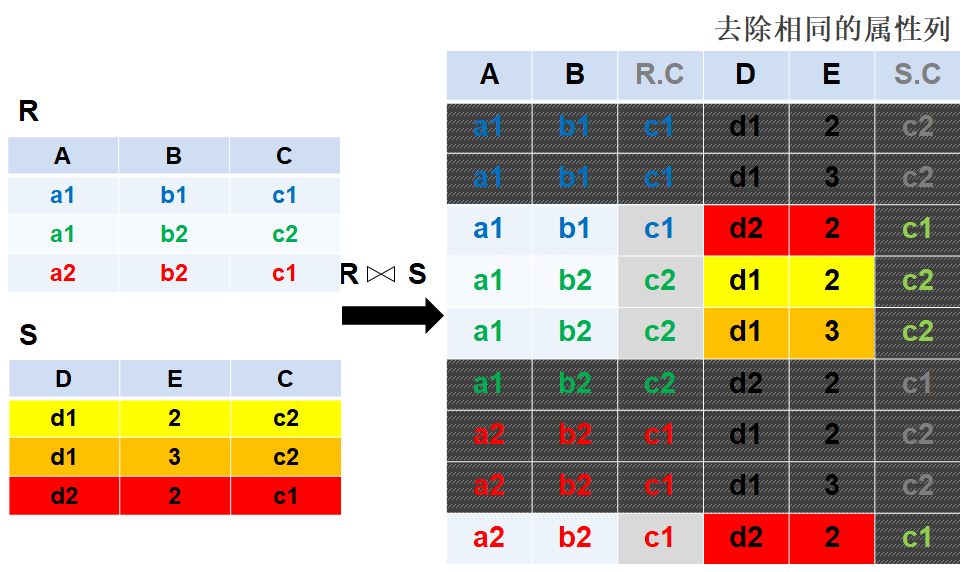除

÷

\div

要求：在满足连接要求的前提下，R表和S表中必须要有相同的属性A（列A）；运算方法：寻找S表中与R表相同的属性组X（列），在R表中寻找匹配S表属性的元组，选取含有S表中属性组X的所有属性的R表元组，再对选出的元组做关于属性X的象集（见上文）得到； *具体算法建议参考 关系代数运算——除法运算_Jack浩2；表达式：

R

÷

S

=

{

i

R

(

X

)

∣

i

R

∈

R

∧

π

Y

(

S

)

⊆

Y

X

}

(

Y

X

=

π

Z

(

σ

X

=

i

R

[

X

]

(

R

)

)

)

.

R\div S=\{i_R(X) | i_R \in R \wedge \pi_Y(S) \subseteq Y_X \}\\(Y_X=\pi_{Z}(\sigma_{X=i_R[X]}(R))).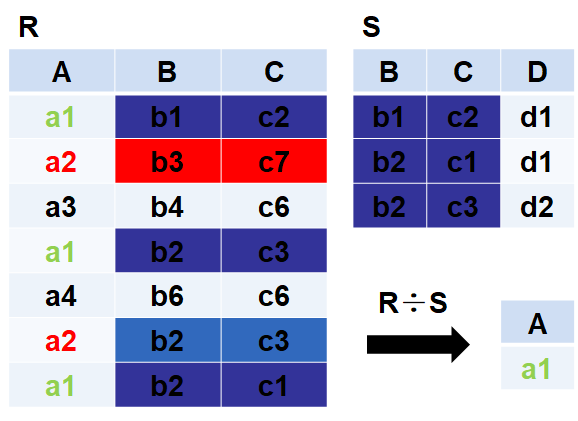以上大部分整理 来源于《数据库系统概论（第5版）》高等教育出版社3，如有问题请不吝赐教，谢谢

Refences

关系代数_百度百科 ↩︎ 关系代数运算——除法运算_Jack浩 ↩︎ 《数据库系统概论（第5版）》_高等教育出版社 ↩︎

展开全文• 总结个等价无穷小相关的关系运算@(微积分)1）f(x)∼g(x)f(x) \sim g(x), g(x)∼h(x)→f(x)∼h(x)g(x) \sim h(x) \rightarrow f(x)\sim h(x) 2）f′(x)→0,g′(x)→0f'(x)\rightarrow 0,g'(x)\rightarrow 0,则f(x)...
总结几个等价无穷小相关的关系运算
@(微积分)
1）

f(x)∼g(x)
$f(x) \sim g(x)$,

g(x)∼h(x)→f(x)∼h(x)
$g(x) \sim h(x) \rightarrow f(x)\sim h(x)$  2）

f′(x)→0,g′(x)→0
$f'(x)\rightarrow 0,g'(x)\rightarrow 0$,则f(x)与g(x)的阶数关系与

f′(x)
$f'(x)$和

g′(x)
$g'(x)$的阶数关系相同。  3）

f′(x)∼Axk⟹f(x)=Ak+1xk+1
$f'(x)\sim Ax^k \Longrightarrow f(x) = \frac{A}{k+1}x^{k+1}$  4）

f(x)∼A(x−a)k,⟹f(x)=Ak+1xk+1
$f(x)\sim A(x-a)^k,\Longrightarrow f(x) = \frac{A}{k+1}x^{k+1}$  5）f(x)是x-a的m阶无穷小，g(u)是u的n阶无穷小，则g(f(x))是x-a的mn阶无穷小。
展开全文• 专门的关系运算并（Union）R和S 具有相同的目n（即两个关系都有n个属性） 相应的属性取自同一个域R∪S 仍为n目关系，由属于R或属于S的元组组成 R∪S = { t|t  R∨t S } 差（Difference）R和S 具有相同的目n ...
概述  传统的集合运算 （并，差，交，笛卡尔积）  专门的关系运算
并（Union）
R和S
具有相同的目n（即两个关系都有n个属性）
相应的属性取自同一个域

R∪S
仍为n目关系，由属于R或属于S的元组组成
R∪S = { t|t  R∨t S }

差（Difference）
R和S
具有相同的目n
相应的属性取自同一个域

R - S
仍为n目关系，由属于R而不属于S的所有元组组成
R -S = { t|tR∧tS }

交（Intersection）
R和S
具有相同的目n
相应的属性取自同一个域

R∩S
仍为n目关系，由既属于R又属于S的元组组成
R∩S = { t|t  R∧t S }
R∩S = R –(R-S）

笛卡尔积（Cartesian Product）

R: n目关系，k1个元组
S: m目关系，k2个元组
R×S
列：（n+m）列元组的集合
元组的前n列是关系R的一个元组
后m列是关系S的一个元组
行：k1×k2个元组
R×S = {tr ts |tr R ∧ tsS }

专门的关系运算
先引入几个记号
（1） R，tR，t[Ai]
设关系模式为R(A1，A2，…，An)
它的一个关系设为R
tR表示t是R的一个元组
t[Ai]则表示元组t中相应于属性Ai的一个分量 
（2） A，t[A]， A
若A={Ai1，Ai2，…，Aik}，其中Ai1，Ai2，…，Aik是A1，A2，…，An中的一部分，则A称为属性列或属性组。
t[A]=(t[Ai1]，t[Ai2]，…，t[Aik])表示元组t在属性列A上诸分量的集合。
A则表示{A1，A2，…，An}中去掉{Ai1，Ai2，…，Aik}后剩余的属性组。

（3） tr ts
R为n目关系，S为m目关系。
tr R，tsS， tr ts称为元组的连接。
tr ts是一个n + m列的元组，前n个分量为R中的一个n元组，后m个分量为S中的一个m元组。

（4）象集Zx
给定一个关系R（X，Z），X和Z为属性组。
当t[X]=x时，x在R中的象集（Images Set）为：
Zx={t[Z]|t R，t[X]=x}
它表示R中属性组X上值为x的诸元组在Z上分量的集合

连接
1）连接也称为θ连接
2）连接运算的含义
从两个关系的笛卡尔积中选取属性间满足一定条件的元组
R         S = {          | tr  R∧ts S∧tr[A]θts[B] }

A和B：分别为R和S上度数相等且可比的属性组
θ：比较运算符
连接运算从R和S的广义笛卡尔积R×S中选取（R关系）在A属性组上的值与（S关系）在B属性组上值满足比较关系θ的元组

3）两类常用连接运算
等值连接（equijoin）
什么是等值连接
θ为“＝”的连接运算称为等值连接
等值连接的含义
从关系R与S的广义笛卡尔积中选取A、B属性值相等的那些元组，即等值连接为：
R    S = {          | tr R∧ts S∧tr[A] = ts[B] }

自然连接（Natural join）
自然连接是一种特殊的等值连接
两个关系中进行比较的分量必须是相同的属性组（同名同域:必须具有相同的属性名，并且出自相同的域集）
在结果中把重复的属性列去掉
自然连接的含义
R和S具有相同的属性组B
R   S = {         | tr R∧ts S∧tr[B] = ts[B] }
一般的连接操作是从行的角度进行运算。
自然连接还需要取消重复列，所以是同时从行和列的角度进行运算。

外连接
在做自然连接时，如果把舍弃的元组也保存在结果关系中，而在其他属性上填空值(Null)，这种连接就叫做外连接（OUTER JOIN）。
左外连接
在做自然连接时，如果只把左边关系R中要舍弃的元组保留就叫做左外连接(LEFT OUTER JOIN或LEFT JOIN)
右外连接
在做自然连接时，如果只把右边关系S中要舍弃的元组保留就叫做右外连接(RIGHT OUTER JOIN或RIGHT JOIN)。

除（Division）
给定关系R (X，Y) 和S (Y，Z)，其中X，Y，Z为属性组。
R中的Y与S中的Y可以有不同的属性名，但必须出自相同的域集。
R与S的除运算得到一个新的关系P(X)，
P是R中满足下列条件的元组在 X 属性列上的投影：
元组在X上分量值x的象集Yx包含S在Y上投影的集合，记作：
R÷S = {tr [X] | tr  R∧πY (S)  Yx }
Yx：x在R中的象集，x = tr[X]

在关系R中，A可以取四个值{a1，a2，a3，a4}
a1的象集为 {(b1，c2)，(b2，c3)，(b2，c1)}
a2的象集为 {(b3，c7)，(b2，c3)}
a3的象集为 {(b4，c6)}
a4的象集为 {(b6，c6)}
S在(B，C)上的投影为
{(b1，c2)，(b2，c1)，(b2，c3) }
只有a1的象集包含了S在(B，C)属性组上的投影
所以     R÷S ={a1}

展开全文数据库 union 属性
• 关系代数是一抽象的查询语言，它用对关系运算来表达查询，其运算对象是关系运算结果亦为关系关系代数的运算符有两类：集合运算符和专门的关系运算符。传统的集合运算是从关系的“水平”方向即行的角度进行，...数据库系统原理 笛卡儿积
• C++实现几种基本的矩阵运算，包含头文件直接调用
• 包括：加法、减法、乘法、除法、乘方、开方等几种运算形式。 其中加减为一级运算，乘除为二级运算，乘方、开方为三级运算。在一道算式中，如果有几级运算存在，则应先进行高级运算，再进行低一级的运算。如：3+22×4...
• 关系代数中，个人觉得比较容易搞混的有这两个概念：传统集合运算和专门关系运算； 传统集合运算：并(∪)、交(∩)、差(—)、笛卡尔积(×)； 专门关系运算：选择(σ)、投影(Π)、连接(∞)、除(÷)； 传统的集合运算将...数据库
• 二进制信息最基本的三逻辑运算：1、逻辑加法(又称“或”)运算，通常用符号“+”或“∨”来表示；2、逻辑乘法(又称“与”)运算，通常用符号“×”或“∧”或“·”来表示；3、逻辑否定(又称“非”)运算。本教程操作...
• Mathematica提高运算速度的几种方法 看到一篇很好的文章，关于提高mma运算速度的，拿出来，讲一下我觉得重要的 这里也是转载翻译的 http://tieba.baidu.com/p/2186436530 下面这个是原文 ...
• A：逻辑运算， and or not xor等等，有专用电路直接...C：关系运算， &gt; &lt; = &gt;= &lt;= &lt;&gt;等等，也有专用电路（数字比较器）可以实现，运算速度也是比较快的。 D...
• ## 关系代数运算——除法运算

万次阅读 多人点赞 2015-09-11 19:20:20
引言  最近在复习数据库的一些基本知识，在学习关系代数运算的...识，现在再次遇到了，就好好的研究了一下，下面给大家分享两种运算方法，希望能给广大读者带来帮助：  第一种：传统的解法  解题过程：数据库 计算
• ## numpy的几种运算

千次阅读 2018-07-24 15:55:32
矩阵的转置有两表示方法 import numpy as np A=np.arange(1,10).reshape(3,3) print(A) print(np.transpose(A)) print(A.T) 输出结果 [[1 2 3] [4 5 6] [7 8 9]] [[1 4 7] [2 5 8] [3 6 9]] [[1 4 7] ...code
• 　另外，还有几种扩充的关系代数操作：外联接(左外联接和右外联接)、外部并和半联接。 　2.除法定义的理解 　设两个关系R和S的元数分别为r和s(r>s>0)，那么R÷S是一个(r-s)元的元组的集合。(R÷S)是满足下列条件的...数据库
• 往往要求根据某个指定的条件是否满足来决定执行的内容。例如，购物在1000元以下的打九五折，1000元及以上的打九折。 C++提供if语句来实现这种条件选择。...关系运算关系表达式 上面if语句中的“amount
•powershell
• ## 关系代数基本运算

千次阅读 2016-02-16 15:07:14
前言：关系代数名称的由来是因为其中含有操作符和操作数，操作数为表，操作符为交、并等。关系代数有分为基于集合的关系代数...此计算需要使得运算的两个表具有相同的字段。例如S1-S2是在S1中而不在S2中的记录的集合笛卡尔积
• 关系数据库关系数据模型关系是一个数学概念。 当把关系的概念引入到数据库系统作为数据模型的数据结构时，既有所限定和也有所扩充。 关系的数学定义例： 课程={离散,C语言…..}，学生={张三,李四…..} 笛卡儿积...数据库
• 一、关系数据结构及形式化定义 1、关系 关系模型的数据结构非常简单，只包含单一的数据结构——关系。... 笛卡儿积是域上的一集合运算。 定义：给定一组域D1，D2，...，Dn，允许其中某些域是相同的，D...数据库
• 代数运算可以分为哪几类，各有什么意义？ 代数运算可以分为对两幅或两幅以上输入图像的加、减、乘、除运算四大类 加法运算通常用于平均值降噪等多种场合。图像相加一般用于同一场景的多幅图像求平均，以便有效 ...
• ## 关系代数运算——（软考三）

千次阅读 热门讨论 2015-10-09 21:39:35
关系代数：是一抽象的查询语言，用对关系运算来表达 查询。关系代数运算的是关系运算结果亦是关系关系代数的基本关系包括：并、交、差、笛卡尔积、选择、投影、连接、除法运算。由于并、交、差运算很简单...
• a=10 b=20 c=expr $a+$b d=$(($a+$b)) e=$[$a+$b] ((f=$a+$b)) echo $c echo$d echo $e echo$f 四个echo均输出30Shell 加法
• a⋅b=b⋅a(a+b)⋅c=a⋅c+b⋅ca×b=−b×a(a+b)×c=a×c+b×c(a×b)×c=b(a⋅c)−a(b⋅c)a×(b×c)=b(a⋅c)−c(a⋅b) a·b = b·a (a+b)·c = a·c+b·c a×b = - b×a (a+b)×c = a×c+b×c (a×b)×c = b(a·c) - a...
• 一、关系模型 为什么学习关系模型？ 我们可以通过关系模型这种简单的数据结构能够描述出现实世界的实体及实体间的各种联系。 什么是关系模型？ 关系模型的基本假定是所有数据都表示为数学上的关系，就是以集合...数据
• ## 几种运算放大电路

千次阅读 2011-06-15 11:23:00
1、电压跟随器：它是同相比例器的特例。输入电阻极大（比射极跟随器的输入电阻还大）。较多使用。2、反相比例器：（注意，你将反相写成了反向）：电路性能好，较多使用。3、同相比例器：由于有共模信号输入，（单端...
• 1、一个数的绝对值，使用如下： qAbs(y) 2、qCeil：向上取整 qCeil(x); 3、求平方，比如x的平方 qPow(2,x);
• 模幂运算是RSA的核心算法，最直接地决定了RSA算法的性能。针对快速模幂运算这一课题，西方现代数学家提出了大量的解决方案，通常都是先将模幂运算转化为模乘运算c 测试 算法 python
• 专门的关系运算 并（Union） R和S 具有相同的目n（即两个关系都有n个属性） 相应的属性取自同一个域 R∪S 仍为n目关系，由属于R或属于S的元组组成 R∪S = { t|t  R∨t S } 1234567812345678 差...
• ## 关系代数运算除

万次阅读 2012-11-09 09:34:11
关系代数运算之除法运算专题...　另外，还有几种扩充的关系代数操作：外联接(左外联接和右外联接)、外部并和半联接。 　2.除法定义的理解 　设两个关系R和S的元数分别为r和s(r>s>0)，那么R÷S是一个(r-s)元的元组...